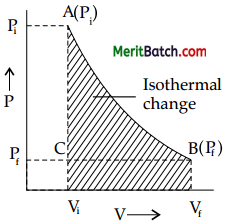Students must start practicing the questions from CBSE Sample Papers for Class 11 Chemistry with Solutions Set 6 are designed as per the revised syllabus.

## CBSE Sample Papers for Class 11 Chemistry Set 6 with Solutions

Time Allowed: 3 hours
Maximum Marks: 70

General Instructions:

• All questions are compulsory.
• This question paper contains 37 questions.
• Questions 1-20 in Section A are objective type-very short answer type questions carrying 1 mark each.
• Questions 21 – 27 in Section B are short answer type questions carrying 2 marks each.
• Questions 28 – 34 in Section C are long-answer 1 type questions carrying 3 marks each.
• Questions 35 – 37 in Section D are long-answer 11 type questions carrying 5 marks each.
• There is no overall choice. However, an internal choice has been provided in six questions of one mark, two questions of two marks, two questions of three marks and two questions of five marks. You must attempt only one of the choices in such questions.
• Use log tables, if necessary. Use of calculator is not allowed.

Section – A

Question 1.
What is the mass percent of carbon in carbon dioxide? 
(A) 0.034%
(B) 27.27%
(C) 3.4%
(D) 28.7%

OR

The empirical formula and molecular mass of a compound are CH2O and 180 g respectively. What will be the molecular formula of the compound?
(A) C9H18O9
(B) CH2O
(C) C6H12O6
(D) C2H4O2
Option (B) is correct.

Explanation:
Molecular mass of CO2 = 12 + 2(16)
= 12 + 32 = 44 g
44 g of CO2 contains 12 g atoms of carbon. Mass percent of carbon
= $$\frac{\text { Mass of carbon in } \mathrm{CO}_2}{\text { Molar mass of } \mathrm{CO}_2}$$ × 100s
= $$\frac{12}{44}$$ × 100
= 27.27%
OR
Option (C) is correct.

Explanation:
Empirical formula mass (CH2O)
= 12 + 2(1) + 16 = 30g
Molecular mass = 180g
n = $$\frac{\text { Molecular mass }}{\text { Empirical formula mass }}$$
= $$\frac{180}{30}$$ = 6

Molecular formula= n × empirical formula
= 6 × CH2O
= C6H12O6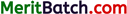Question 2.
On the basis of thermochemical equations (i), (ii) and (iii), find out which of the algebraic relationship given in options (A) to (D) is correct. 
(i) C (graphite) + O2(g) → C02(g); ΔfH = x kj mol-1
(ii) C (graphite) + $$\frac{1}{2}$$O2 (g) → CO(g); ΔrH = y kj mol-1
(iii) CO (g) + $$\frac{1}{2}$$O2 (g) → C02 (g) ;ΔH = z kj mol-1
(A) z = x + y
(B) x = y – z
(C) x = y + z
(D) y = 2z – x
Option (C) is correct.
Explanation: The algebric relationships of the given reaction is Eq.(i) – Eq.(ii) = Eq.(iii)
(i) C(graphite) + O2(g) → CO2(g) ; ΔrH = x kJ mol-1
(ii) C(graphite) + $$\frac{1}{2}$$O2(g) → CO(g) ; ΔrH = V kJ
(iii) CO(g) + $$\frac{1}{2}$$O2(g) → CO2(g) ; ΔrH = z kJ mol-1
Hence, x – y = z or x = y + z

Question 3.
Dipole-dipole forces act between the molecules possessing permanent dipole. Ends of dipoles possess ‘partial charges’. The partial charge is : 
(A) more than unit electronic charge.
(B) equal to unit electronic charge.
(C) less than unit electronic charge.
(D) double the unit electronic charge.
Option (C) is correct.

Explanation:
Partial charge is the small charge developed by displacement of electrons.

Question 4.
The first ionisation enthalpies of Na, Mg, Al and Si are in the order : 
(A) Na < Mg > Al < Si (B) Na > Mg > Al > Si
(C) Na < Mg < Al < Si (D) Na > Mg > Al < Si OR Which of the following is the correct order of size of the given species: (A) I > I > I+
(B) I+ > I > I
(C) I > I+ > I
(D) I > I > I+
Option (A) is correct.

Explanation:
On moving from left to right in a period first ionisation enthalpy increases normally. But, in Mg, as an electron needs to be removed from fully filled s orbital therefore, first ionisation enthalpy of Mg is more than Al.

OR

Option (D) is correct.

Explanation :
Size of cation is smaller while that of anion is bigger than its parent atom.

Question 5.
What will be correct order of vapour pressure of water, acetone and ether at 30°C. Given that among these compounds, water has maximum boiling point and ether has minimum boiling point? 
(A) Water < ether <acetone
(B) Water < acetone < ether
(C) Ether < acetone < water
(D) Acetone < ether < water
Option (B) is correct.

Explanation:
Greater is the boiling point, less is the vapour pressure.Question 6.
Which of the following carbocation is most stable?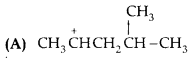(B) (CH3)3C+
(C) CH3CH2+CH2
(D) CH3+CHCH2CH3
Option (B) is correct.

Explanation:
Tertiary carbocation is most stable.

The variables Pressure, Volume, Concentration and Temperature may change the State of Equilibrium. The change is governed by the Le-Chatelier’s principle. The decomposition of NH3(g) can be made spontaneous by increasing the temperature and lowering pressure. In the reaction, removal of any product from the reaction mixture makes the reversible reaction irreversible and therefore, reaction proceeds to completion.
Answer the questions (7) to (10) given below:

Question 7.
The equilibrium Solid → Liquid → Gas will shift in forward direction when:
(A) temperature is increased
(B) temperature is lowered
(C) pressure is increased
(D) pressure is lowered
Option (A) is correct.

Explanation:
Increase in temperature will favour the forward reaction which is endothermic.

Question 8.
Change in free energy for the equilibrium, gaseous reaction, PCl5 → PCl3 + Cl2 on addition of an inert gas at constant pressure and at constant volume is respectively:
(A) decrease, no change
(B) increase, no change
(C) no change, no change
(D) no change, decrease
Option (A) is correct.

Explanation:
Addition of inert gas at constant volume has no effect on any equilibrium reaction. When inert gas is added at constant pressure in the given reaction, it proceeds in forward direction. DG° remains constant, but DG decreases as the reaction proceeds spontaneously in forward direction to attain equilibrium.

Question 9.
At 25°C, the equilibrium constant K1 and I(z are for the reactions:Which of the following shows the relation between two equilibrium constants?
(A) K2 = K2
(B) K2 = 1/(K)
(D) K1 = 1/(K)
(D) K1 = 1/K2
Option (C) is correct.

Explanation: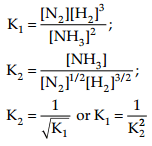Question 10.
A liquid is in equilibrium with its vapour at its boiling point. On an average, the molecules in the two phases have equal:
(A) Intermolecular forces
(B) Potential energy
(C) Kinetic energy
(D) None of these
Option (C) is correct.

Explanation:
At boiling point, liquid and vapour phases both are present. So, the molecules in the two phases have equal kinetic energy.Question 11.
The addition of HCl to an alkene proceeds in two steps. The first step is the attack of W ion to >C =C< portion which can be shown as A I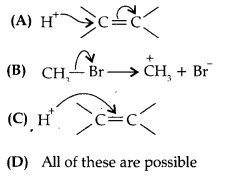Option (A) is correct.

Explanation:
Since double bond is a source of electrons and the charge flows from source of more electron density, therefore, electrons of the double bond attack the proton.

Question 12.
How will you convert: ethyne to but-2-yne.Students directly write the products and forget to mention side products and reaction conditions.

Make a list of important conversions and learn them.
Understand each step and conditions involved during conversion reactions.

Question 13.
The structure of triphenylmethyl cation is given here. This is very stable and some of its salts can be stored for months. Explain the cause of high stability of this cation.Triphenylmethyl cation is a tertiary carbocation which can show nine possible canonical structures and hence is very stable. The three canonical forms for one benzene ring are shown below.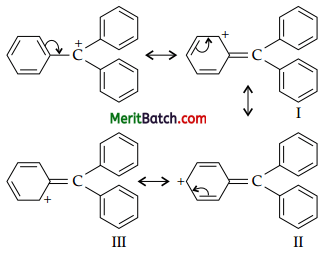Question 14.
Can we separate two liquids A (b.p. 353 K) and B (b.p. 365 K) present in a mixture by simple distillation? 
No, because in simple distillation, vapours of both the liquids will be formed simultaneously and will condense together in receiver as the difference between the boiling points is very less. They can be separated by fractional distillation.

Students sometimes answer in yes or no without giving legit explanation.

The alternate method of separation also must be mentioned.

Question 15.
What do you mean by cracking? 
The thermal decomposition of higher hydrocarbons into lower hydrocarbons in the presence or absence of a catalyst is called cracking.

Question 16.
Assign the oxidation number to Cr in K2Cr2O7.
Let the oxidation number of Cr be x
2 × (+1) + 2x + 7 × (- 2) = 0
2 + 2x – 14 = 0
2x – 12 = 0
x = $$\frac{12}{2}$$ = +6
Oxidation number of Cr in K2Cr2O7 = +6Question 17.
Assertion (A): Toluene on Friedal-Crafts methylation gives o- and p-xylene.
Reason (R): CH3-group bonded to benzene ring increases electron density at o- and p- position.
(A) Both A and R are correct and R is the correct explanation of A.
(B) Both A and R are correct but R is not the correct explanation of A.
(C) Both A and R are not correct.
(D) A is not correct but R is correct.
Option (A) is correct.

Question 18.
Assertion (A): Benzene on heating with cone. H2SO4 gives benzene sulphonic acid which when heated with superheated steam under pressure gives benzene. 
Reason (R): Sulphonation is a reversible process.
(A) Both A and R are correct and R is the correct explanation of A.
(B) Both A and R are correct but R is not the correct explanation of A.
(C) Both A and R are not correct.
(D) A is not correct but R is correct.
Option (A) is correct.

Explanation:
Sulphonation of benzene is an electrophilic substitution reaction in which SO3 acts as the electrophile.

Question 19.
Why there is large number of lines in hydrogen spectrum? 
Large number of lines are there in hydrogen spec¬trum because different possible transitions can be observed which leads to large number of spectral lines.

Question 20.
Pressure is determined as force per unit area of the surface. The SI unit of pressure, pascal is as shown below:
1 Pa = 1 Nm-2
If mass of air at sea level is 1034 g cm-2, calculate the pressure in pascal. 
Mass of air at sea level = 1034 g cm-2
Acceleration due to gravity, g = 9.8 ms-2
Pressure is the force or weight per unit area.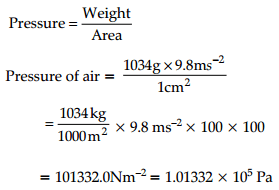Section – B

Question 21.
What does the following prefixes stand for
(a) pico
(b) nano
(c) micro
(d) deci
OR
Write main points of Dalton’s atomic theory. 

 Prefixe Stand for (a) pico (p) 10-12 (b) nano (n) 10-9 (c) micro (μ) 10-6 (d) deci (d) 10-1

OR
The main points of Dalton’s atomic theory are:
(i) All matters are made of atoms. Atoms are indivisible and indestructible.
(ii) All atoms of a given element are identical in mass and properties. Atoms of different elements differ in mass.
(iii) Compounds are formed when atoms of different elements combine in a fixed ratio.
(iv) Chemical reactions involve reorganisation of atoms. These are neither created nor destroyed in a chemical reaction.Question 22.
Chlorophyll present in green leaves of plants absorbs light at 4.620 × 1014Hz. Calculate the wavelength of radiation in nanometer. Which part of the electromagnetic spectrum does it belong to? 
Given, v = 4.620 × 1014 Hz
λ = ?
Wavelength λ = $$\frac{c}{v}$$
= 0.6494 × 10-6 m
= 649.4 × 10-9 m
= 649.4 nm
It belongs to visible region.

Question 23.
In both water and diethyl ether, the central atom viz. O-atom has same hybridisation. Why do they have different bond angles? Which one has greater bond angle? 
Both water and diethyl ether have the central atom O in sp3 hybrid state with two lone pairs of electronsBut due to the greater repulsion between two ethyl (C2H5) groups in diethyl ether than between two H-atoms in H2O result in greater bond angle (110°) in diethyl ether than 104.5° in that of water (H2O).

Question 24.
(a) Why is an organic compound fused with sodium for testing nitrogen, halogens and sulphur?
(b) Under what conditions can the process of steam distillation used? 
(i) On fusing with sodium metal, the elements present in an organic compound are converted from covalent form into the ionic form.
(ii) Steam distillation is used to purify the substances which are steam volatile and water and the liquid are not miscible with each other.

Question 25.
Write the name of the isomerism shown by the following pairs:
(i) Buta-1,3-diene and But-l-yne (C4H6)
(ii) Ethoxy butane and Propoxy propane (C6H14O). 
OR
Which bond is more polar in the following pairs of molecules.
(i) H3C-H, H3C-Br
(ii) H3C-NH2, H2C-OH
(i) Functional isomerism
H2C=CH – CH = CH2 and HC = C-CH2CH3

(ii) Metamerism
CH3 – CH2 – CH2 – CH2 – O- CH2CH3 and C3H7OC3H7

Students get confuse and give wrong answers.

Understand different type of isomerism with examples, different functional groups too.
OR
(i) C-Br because Br is more electronegative than H.
(ii) C-O because O is more electronegative than N.

Question 26.
An alkane C8H18 is obtained as the only product on subjecting a primary alkyl halide to Wurtz reaction. On
monobromination this alkane yields a single isomer of a tertiary bromide. Write the structure of alkane and the tertiary bromide.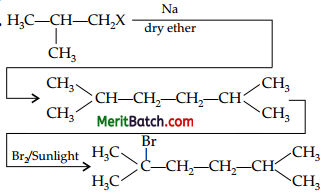Question 27.
What are relative stabilities of different conformations of ethane? Is is possible to isolate these at room temperature? 
Staggered form of ethane is more stable than the eclipsed form because the force of repulsion between hydrogen atoms on adjacent C atom is minimum. The energy difference between the staggered form and the eclipsed form of ethane is just 12.55 kJ mol- 1. Therefore, it is not possible for these two forms of ethane to isolate at room temperature.

Section – C

Question 28.
What are (a) representative elements (b) transition elements (c) Lanthanoid and actinoids. Give their position in modern periodic table. 
(a) Representative Elements : Group 1, 2, 13, 14, 15, 16, 17 and 18 exhibit the main groups of the periodic table and so, the elements of these groups are collectively called representative elements.
These elements belong to s-block and p-block in modern periodic table.

(b) Transition Elements : d-block transition metals form a bridge between the chemically active metals of s-block elements and the less active elements of Groups 13 and 14 are known as transition elements.

(c) Lanthanoids and Actinoids : The elements of 4f series [i.e., from Ce (Z = 58) to Lu (Z = 71)] are called lanthanoids and the elements of 5f series [i.e., from Th (Z = 90) to Lr (Z = 103)] are called actinoids. These elements belong to /-block elements in the modern periodic table which lie at the bottom of the periodic table.Question 29.
The Mn3+ ion is unstable in solution and undergoes disproportionation to give Mn2+, MnO2 and H+ ion. Write a balanced ionic equation for the reaction. 
The skeletal ionic equation is,
Mn3+(aq) → Mn2+(aq) + MnO2(s) + H+(aq)

Reduction half reaction
Mn3+(aq) + e → Mn2+

Oxidation half reaction
Mn3+(aq)→ MnO2 + e

Balance charge by adding 4H+ to right side and then balance O atoms by adding 2H2O to left side in oxidation half reaction
Mn3+(aq) + 2H2O(l) → MnO2(s) + e + 4H+(aq)
By adding both equations, we get
2Mn3+(aq) + 2H2O(l) → Mn2+ + MnO2(s) + 4H+(aq)
This represents the balanced redox reaction (disproportionation reaction).

Question 30.
Calculate the standard enthalpy of formation of CH3OH(l) from the following data :
(i) CH3OH(l) + $$\frac{3}{2}$$02(g) → C02(g)+ 2H2O(l) ΔrH° = – 726 kj/mol
(ii) C(s) + 02(g) → C02(g); ΔcH° = – 393 kj/mol
(iii) H2(g) + ½O2(g) → H2O(l); ΔfH° = – 286 kj/mol. 
The required equation is
C(s) + 2H2(g) + $$\frac{1}{2}$$ O2(g) → CH3OHO);
ΔfH° = ± ?
To get the above required equation :
Step 1 : Multiply eq. (iii) by 2 and add to eq. (ii)
C(s) + 2H2(g) + 2O2(g) → CO2(g) + 2H2O(l) ……………(iv)
ΔfH° = (2 x – 286) + (- 393)
= – 572 – 393
= – 965 kJ/mol

Step 2 : Subtract eq. (i) from eq. (iv)
C(s) + 2H2(g) + $$\frac{1}{2}$$O2(g) → CH3OH(l);
ΔH = – 965 – (- 726)
= – 239 kJ/mol
ΔfH° = – 239 kJ/mol

Question 31.
(i) State the formula and name of the conjugate base of each of the following acids :
(a) H3O+
(b) HSO4
(c) NH4+
(d) HF
(e) CH3COOH
(f) CH3NH3+
(g) H3PO4
(h) H2PO4

(ii) The ionic product of water is 0.11 × 10-14 at 273 K, 1 × 10-14 at 298 K and 7.5 × 10-14 at 373K. Deduce from this data whether the ionization of water to hydrogen and hydroxide ion is exothermic or endothermic. 
(i) The formula and name of the conjugate base are:
(a) H3O+: Water
(b) SO42- : Sulphate ion
(c) NH3 : Ammonia
(d) F : Fluoride ion
(e) CH3COO : Acetate ion
(f) CH3NH2 : Methylamine
(g) H2PO4 : Dihydrogen phosphate
(h)H2PO42- : Mono hydrogen phosphate

(ii) Kw = [H3O+] [OH]
According to the data, the value of K, is increasing with temperature. Therefore, according to Le- Chateliebs principle, the ionisation of water is endothermic.Question 32.
Explain:
(i) Tea or coffee is sipped from the saucer, when it is quite hot.
(ii) Liquids possess fluidity. 
(i) Tea or coffee is sipped from the saucer, when it is quite hot because it has larger surface area than the cup. In larger surface area, the rate of evaporation is faster due to which tea or coffee cools rapidly.
(ii) Liquids have indefinite shape. They take the shape of the container in which they are placed. This is due to the fact that the molecules of liquids are in a state of constant random motion and therefore they can move freely. So, the liquids possess fluidity.

Question 33.
(a) The effect of uncertainty principle is significant only for motion of microscopic particles and is negligible for the macroscopic particles. Justify the statement with the help of a suitable example.
(b) What is the difference between the terms orbit and orbital ? 
If mass of an object = 1 mg = 10-6 kg
Then, according to Heisenberg’s uncertainty principle,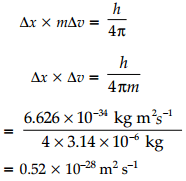Since, the value of Δx.Δv obtained is very small and is insignificant. So, effect of uncertainty principle is significant only for motion of microscopic particles and is negligible for the macroscopic particles.
(b)

 Orbit Orbital 1. An orbit is well defined circular path around the nucleus in which the electrons revolve. 1. An orbital is the three­dimensional space around the nucleus within which the prob­ability of finding an electron is maximum (upto 90%). 2. It represents the planar motion of an electron around the nucleus. 2. It represents the three dimensional motion of an electron around the nucleus.

Question 34.
Calculate the number of moles:
(i) 5.0 L of 0.75 M Na2CO3
(ii) 7.85 g of Fe
(iii) 34.2 g of sucrose (C12H22O11) 
OR
A compound made up of two elements A and B has A = 70%, B= 30%. Their relative number of moles in the compound is 1.25 and 1.88, calculate:
(i) Atomic masses of the elements A and B.
(ii) Molecular formula of the compound, if its molecular mass is found to be 160.
(i) Number of moles of Na2 CO3 = Molarity × Volume of solution in litre
= 0.75 × 5
= 3.75 mol

(ii) Number of moles of Fe
= $$\frac{\text { Mass }}{\text { Molecular mass }}$$
= $$\frac{34.2}{342}$$
= 0.14

(iii) Number of moles of sucrose
= $$\frac{\text { Mass }}{\text { Molecular mass }}$$
= $$\frac{34.2}{342}$$
= 0.1

OR

(i) Atomic mass of element A
= $$\frac{\% \text { of element } \mathrm{A}}{\text { Relative number of moles }}$$
= $$\frac{70}{1.25}$$
= 56

Atomic mass of element B
= $$\frac{\% \text { of element } \mathrm{B}}{\text { Relative number of moles }}$$
= $$\frac{30}{1.88}$$
= 15.957 ≈ 16
(ii)

 Compound Simplest molar ratio Simplest whole-number ratio A $$\frac{1.25}{1.25}$$= 1 2 B $$\frac{1.88}{1.25}$$= 15 3

Empirical formula of compound = A2B3
Molecular mass = 160
Empirical formula mass
= 2(56) + 3(16)
= 112 + 48 = 160

n = $$\frac{\text { Molecular mass }}{\text { Empirical formula mass }}$$
= $$\frac{160}{160}$$ = 1

Molecular formula
= n × Empirical formula
= 1 × A2B3
= A2B3

Sometimes students forget to multiply by n while finding molecular formula and do mistakes.

Understand the problem and check for the necessary data, which are available in the problem. Follow steps and ensure no steps are missed.Section – D

Question 35.
(i) For each of the following compounds, write a more condensed formula and also their bond-line formulae.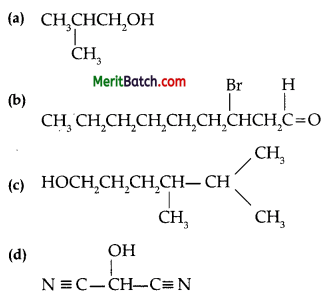(ii) Write the I.U.RA.C. name of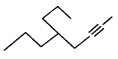OR
(a) A sample of 0.50 g of an organic compound was treated according to Kjeldahl’s method. The ammonia evolved was absorbed in 50 ml of 0.5 M H2SO4. The residual acid required 60 mL of 0.5 M solution of NaOH for neutralisation. Find the percentage composition of nitrogen in the compound.
(b) In the estimation of sulphur by Carius method, 0.468 g of an organic sulphur compound afforded 0.668 g of barium sulphate. Find out the percentage of sulphur in the given compound.
(i) Condensed formulae
(a) (CH3)2CHCH2OH
(b) CH3(CH2)5CHBrCH2CHO
(c) HO(CH2)3CH(CH3)CH(CH3)2
(d) HOCH(CN)2

Bond-line formulae-Sometimes students ignore the triple bond in (ii) question.

While writing bond-line formula, observe carefully. It is similar to structural formula.

OR

Given that, total mass of organic compound = 0.50 g 60 mL of 0.5 M solution of NaOH was required by residual acid for neutralisation.
60 mL of 0.5 M NaOH solution = $$\frac{60}{2}$$mL of 0.5 M
H2SO4 = 30 mL of 0.5 M H2SO4
Acid consumed in absorption of evolved ammonia is (50-30) mL = 20 mL
Again, 20 mL of 0.5 M
H2SO4 = 40 mL of 0.5 M NH3
Also, since 1000 mL of 1 M NH3 contains 14 g of nitrogen,
40 mL of 0.5 M NH3 will contains = $$\frac{14 \times 40}{1000}$$ × 0.5
= 0.28 g of N
Therefore, percentage of nitrogen in 0.50 g of organic compound = $$\frac{0.28}{0.50}$$ × 100
= 5.6%

Students forget steps and end up with mistakes.

Understand the problem and practice steps wise and do calculations carefully.

(b) Given, total mass of organic compound = 0.468 g Mass of barium sulphate formed = 0.668 g
1 mol of BaSO4 = 233 g of BaSO4 = 32 g of sulphur
Thus, 0.668 g of BaSO4 contains $$\frac{32 \times 0.668}{233}$$ g of
sulphur = 0.0917 g of sulphur

Therefore, percentage of sulphur = $$\frac{0.0917}{0.468}$$ × 100
= 19.59 %
Hence, the percentage of sulphur in the given compound is 19.59 %.

Question 36.
Give mechanism of addition of HBr to Propene. 
Addition of HBr to propene (unsymmetrical alkene) follows Markovnikov’s rule according to which the negative part of the addendum gets attached to that C atom which possesses lesser number of hydrogen atoms.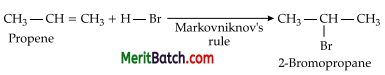Mechanism: Hydrogen bromide provides an electrophile, H+, which attacks the double bond to form carbocation as:Secondary carbocations are more stable than primary carbocations. Therefore, the former predominates as it will form at a faster rate. Thus, in the next step, Br attacks the carbocation to form 2-bromopropane as the major product.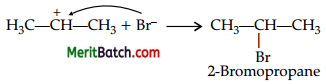Addition of HBr to unsymmetrical alkenes like propene in the presence of light or peroxide takes place contrary to the Markovnikov’s rule. This so happens only with HBr but not with HCl and HI. This addition of HBr to propene in the presence of benzoyl peroxide follows anti-Markovnikov’s rule or peroxide effect or Kharasch effect.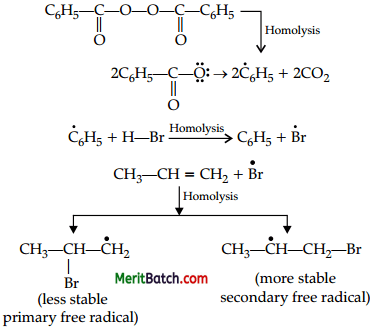Secondary free radicals are more stable than primary radicals. Therefore, the former predominates since it forms at a faster rate. Thus, 1-bromopropane is obtained as the major product.• Some students write the reaction, but forget to explain the mechanism.
• Some students forget to explain the attack of Br- on carbocation.

Students must explain addition using Markovnikov’s rule as well as anti – Markovnikov’s rule.Question 37.
Derive the relationship between AH and AU for an ideal gas. Explain each term involved in the equation. 
OR
Graphically show the total work done in an expansion when the state of an ideal gas is changed reversibly and isothermally from (pi, Vi to (pf Vf). With the help of a pV plot, compare the work done in the above case with that carried out against a constant external pressure pf.
According to first law of thermodynamics,
q = ∆U + W
= ∆U + p∆V
At constant volume, ∆V = 0
qv = ∆U
where qv = Heat absorbed at constant volume
∆U = Change in internal energy
Similarly qp = DH
where qp = Heat absorbed at constant pressure
DH = Enthalpy change of the system
Since, the enthalpy change of a system is equal to heat absorbed or heat evolved by the system at constant pressure.
Now, at constant pressure
∆H = ∆U + p∆V
∆V = change in volume
∆H = ∆U + p(Vf – Vi)
∆H = ∆U + (pVf – pVi) …(i)
Vi = initial volume of the system
Vf = Final volume of the system

For ideal gases,
pV = nRT
pVi = nRT
and pVf = npRT
where nr = number of moles of the gaseous reactants
np = number of moles of the gaseous products

Equation (i) becomes,
∆H = ∆U + (npRT – nrRT)
= ∆U + (np – nr) RT
or ∆H = ∆U + ∆ngRT
where ∆ng = Difference between the number of moles of the gaseous products and reactants.

Students miss steps and explanations and thus lose marks.

Understand the derivation step by step and practice thoroughly. Explain all the terms involved in the equations.

OR

(i) Reversible work is represented by the combined areas ABC and BCViVf.
(ii) Work against constant pressure, pf is represented by the area BCViVf .
Work (i) > Work (ii)